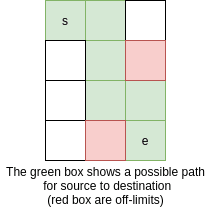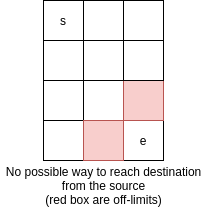Home Practice Programming Find if a path is possible for reaching bottom right from the...

# Find if a path is possible for reaching bottom right from the top left in a nxm grid

A robot sitting on the upper left corner(0,0) of a grid with r rows and c columns.
The robot can only move in two directions: right or down, but certain cells are “off-limits” such that the robot cannot step on them. Design an algorithm to find if there exists a path for the robot for reaching the bottom right from the top left.

The problem requires an understanding of Dynamic Programming. Read here…The first grid has many possible paths of reaching destination(e) form source(s) and one of them is shown using green. In the second grid, there is no possible path to reach the destination from the source.

#### Algorithm

1. Let’s start from the end(r,c) and start walking in up(r-1,c) and left(r,c-1) direction.
2.  If we encounter an off-limit, we will take the alternate path.
3. If both the path contains the off-limit, stop returning no possible path.
4. Repeat the above steps until we reach the source and return true(path is possible).

Since the subproblems involve repetition, Dynamic Programming is used to speed up and reduce time complexity.

##### Implementation of the above algorithm in CPP
```#include <bits/stdc++.h>
using namespace std;

int steps_possible(int r, int c, vector<vector<int> >&dp){

if(r<0 ||c<0 ||dp[r]==-2)
return 0;

if(r==0 && c==0)
return 1;

if(dp[r]==-1)                         //we are visiting the current grid first time
dp[r] = steps_possible(r-1,c,dp) || steps_possible(r,c-1,dp);

return dp[r];
}

int main()
{
int r=4,c=3;
vector<vector<int> >dp(r,vector<int>(c,-1));

dp=-2;     // offlimit(invalid) grid
dp=-2;     // offlimit(invalid) grid
dp=-2;     // offlimit(invalid) grid

int res = steps_possible(r-1,c-1,dp);
cout<<boolalpha<<bool(res)<<endl;

return 0;
}
```

In the above program, the dp[i][j] is used to store the values of subproblems. It is assigned -1, which tells that the current step/subproblem is not computed and -2, tells that it is invalid of the off-limit grid.

```Output
true```

#### Time Complexity

The time complexity of the above program is O(RC), where R is the number of Rows(r) and C is the number of Columns(c) using Dynamic Programming.